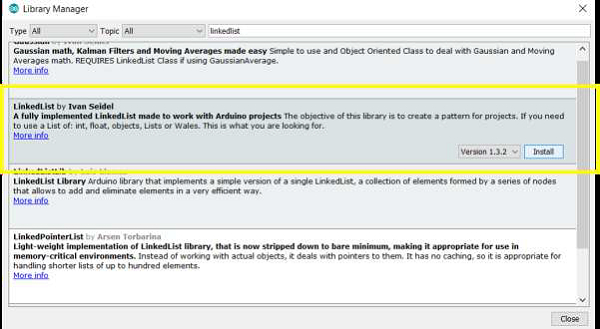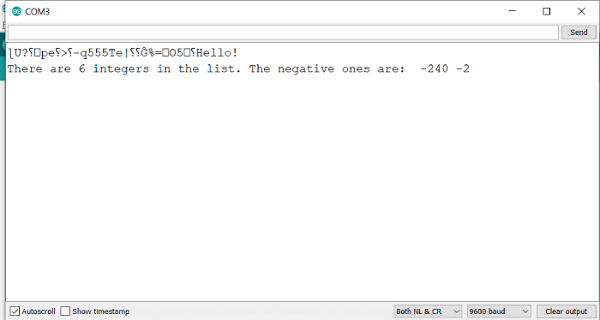The LinkedList library by Ivan Seidel helps implement this data structure in Arduino. A linked list contains a set of nodes wherein each node contains some data, and a link (reference) to the next node in the list.

To install this library, go to Library Manager, and search for LinkedList.Once installed, go to: File→ Examples→LinkedList and open the SimpleIntegerList example.

Much of the code is self-explanatory. We include the library and create the object, specifying integer as the data type.

#include <LinkedList.h>
LinkedList<int> myList = LinkedList<int>();

Within setup, we populate the list with some integers, using the .add() function.

void setup()
{
Serial.begin(9600);
Serial.println("Hello!");

// Add some stuff to the list
int k = -240,
l = 123,
m = -2,
n = 222;
}

In the loop, we print the size of the list, using the .size() function, and get each successive element using the .get() function. If the value of that element is less than 0, we print it.

void loop() {
int listSize = myList.size();

Serial.print("There are ");
Serial.print(listSize);
Serial.print(" integers in the list. The negative ones are: ");

// Print Negative numbers
for (int h = 0; h < listSize; h++) {

// Get value from list
int val = myList.get(h);

// If the value is negative, print it
if (val < 0) {
Serial.print(" ");
Serial.print(val);
}
}

while (true); // nothing else to do, loop forever
}

## Output

When run on the Serial Monitor, the output is −As you can see, this one is quite straightforward. You are encouraged to go through the other examples that come in with this library as well.